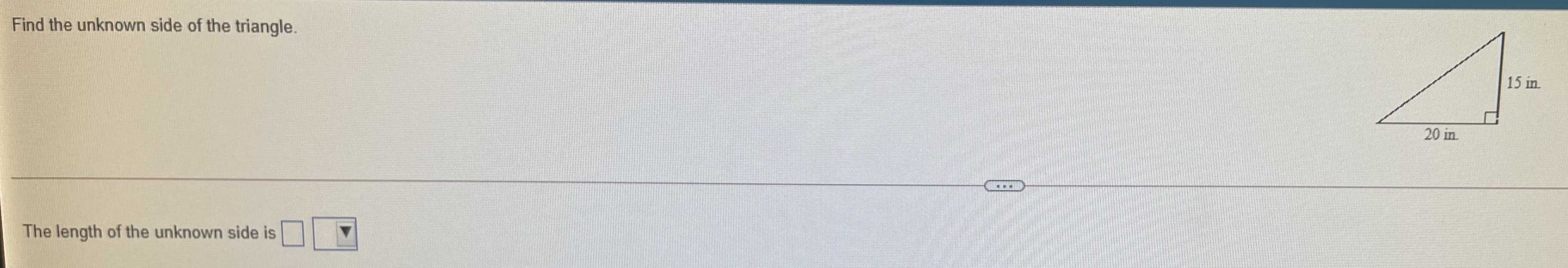### Still have math questions?Find the unknown side of the triangle. The length of the unknown side is $$\square$$
$$= \sqrt{20^{2} + 15^{2} } = 25$$